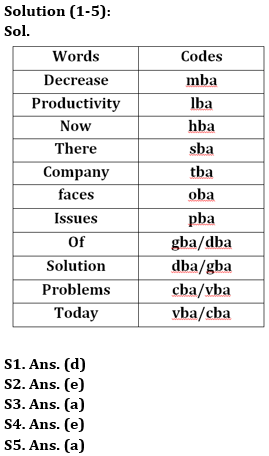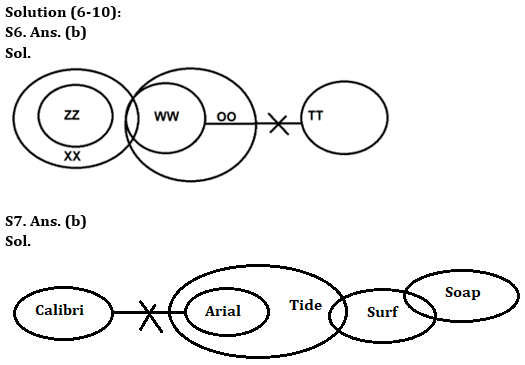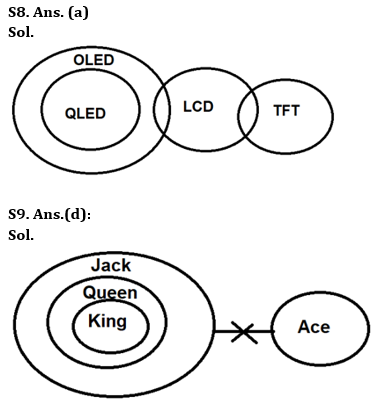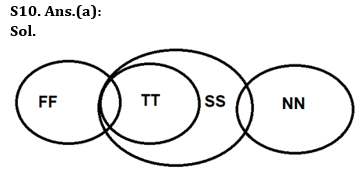Latest Banking jobs   »

# Reasoning Quizzes Quiz For IRDA AM 2023-16th May

Directions (1-5): Answer the questions based on the information given below.
In a certain language,
‘Decrease productivity now there’ is coded as ‘Iba sba mba hba’
‘Company there faces issues’ is coded as ‘oba sba tba pba’
‘Decrease issues of solution’ is coded as ‘dba pba mba gba’
‘Productivity faces problems today’ is coded as ‘vba Iba cba oba’

Q1. Which of the following is the code for ‘company’?
(a) dba
(b) pba
(c) oba
(d) tba
(e) None of these

Q2. Which of the following words is coded as ‘gba’?
(a) Of
(b) Situation
(c) Now
(d) There
(e) Either ‘of’ or ‘situation’

Q3. Which of the following is coded as ‘sba’?
(a) There
(b) Now
(c) Productivity
(d) Either ‘there’ or ‘now’
(e) Now

Q4. What is the code of the words ‘Productivity today’?
(a) hba vba
(b) lba vba
(c) Iba cba
(d) cba hba
(e) Either (b) or (c)

Q5. What is the code of the word ‘Issues’?
(a) pba
(b) hba
(c) tba
(d) lba
(e) None of these

Directions (6-10): In the question below, four statements are given followed by two conclusions numbered I and II. You have to take the given statements to be true even if they seem to be at variance with commonly known facts. Read all the conclusions and then decide which of the given conclusions logically follows from the given statements disregarding commonly known facts.

Q6. Statements: All ZZ is XX.
A few XX is WW.
Each WW is OO.
No TT is WW.
Conclusions: I. A few ZZ is OO
II. All XX being OO is a possibility
(a) If only conclusion I follows
(b) If only conclusion II follows
(c) If either conclusion I or conclusion II follows
(d) If both conclusions I and II follow
(e) If neither conclusion I nor conclusion II follows

Q7. Statements: No Calibri is Arial.
All Arial is Tide.
Only a few Tides is Surf.
Some Surf is Soap.
Conclusions: I. A few Tides is Calibri
II. All Surf being Tide is a possibility
(a) If only conclusion I follows
(b) If only conclusion II follows
(c) If either conclusion I or conclusion II follows
(d) If both conclusions I and II follow
(e) If neither conclusion I nor conclusion II follows

Q8. Statements: Only OLED is QLED.
Some LCD is OLED.
Some TFT are LCD.
Conclusions: I. Some OLED are QLED
II. Some OLED are TFT
(a) If only conclusion I follows
(b) If only conclusion II follows
(c) If either conclusion I or conclusion II follows
(d) If both conclusions I and II follow
(e) If neither conclusion I nor conclusion II follows

Q9. Statements: All King are Queen.
Each Queen is Jack.
No Ace is Jack.
Conclusions: I. Some Jack is King
II. No Queen is Ace
(a) If only conclusion I follows
(b) If only conclusion II follows
(c) If either conclusion I or conclusion II follows
(d) If both conclusions I and II follow
(e) If neither conclusion I nor conclusion II follows

Q10. Statements: Only a few FF is TT.
All TT is SS.
Only a few SS is NN.
Conclusions: I. All NN being SS is a possibility
II. Mostly FF is NN
(a) If only conclusion I follows
(b) If only conclusion II follows
(c) If either conclusion I or conclusion II follows
(d) If both conclusions I and II follow
(e) If neither conclusion I nor conclusion II follows

Solutions## FAQs

### How many sections are there in the IRDAI exam?

There are 4 sections in the IRDAI assistant manager online preliminary exam. They are Reasoning, English Language, General awareness, Quantitative aptitude.

#### Congratulations!Union Budget 2023-24: Free PDF